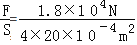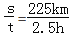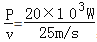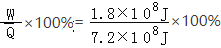# 小洁家购买某型号的四轮汽车的质量为1.8t，每个轮子与地面的接触面积为

1）汽车静止时对路面的压强．

2）汽车行驶的平均速度是多少？

3）汽车行驶过程的牵引力是多大？

4）汽车发动机的效率是多少？（已知ρ汽油=0.8×103kg/m3q汽油=4.5×107J/kgg=10N/kg

1条回答
• 解析：

1）汽车静止时对路面的压力：

F=G=mg=1.8×103kg×10N/kg=1.8×104N

汽车对地面压强：

p==2.25×106Pa

2）汽车行驶速度：

v==90km/h=25m/s

3汽车牵引力

F==800N

4）汽车行驶过程所做的功：

W=Pt=20×103W×2.5×3600s=1.8×108J

根据ρ=可得：

消耗的燃油的质量

m=ρV=0.8×103kg/m3×20×103m3=16kg

燃油完全燃烧放出热量：

Q=mq=4.5×107J/kg×16kg=7.2×108J

汽车发动机的效率是：

η==25%

故：

1）汽车静止时对路面的压强为2.25×106Pa

2）汽车行驶速度是25m/s

3）汽车行驶过程的牵引力是800N

4）汽车发动机的效率是25%

讨论(1)看不清?